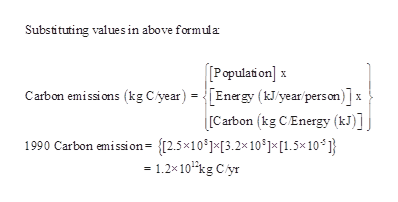# Environmental Engineering, disaggregation exponential growth question:Consider the following disaggregation of carbon emissions:Carbon emissions (kg C/year)= [Population] x [Energy (kJ/year/person)] x [Carbon (kg C/Energy (kJ)]Using the following estimates for the United States and assuming growth rates remain constant, 1990 amounts:Population: 2.5 x 108, growth rate: 0.6%Energy: 3.2 x 108 kJ/yr/person, growth rate: 0.5%Carbon emissions: 1.5 x 10-5 kg C/kJ, growth rate: -0.3%Find:a) The carbon emission rate in 2020b) The carbon emitted in those 30 yearsc) The total energy demand in 2020d) the per capita carbon emission rate in 2020

Question
63 views

Environmental Engineering, disaggregation exponential growth question:

Consider the following disaggregation of carbon emissions:

Carbon emissions (kg C/year)= [Population] x [Energy (kJ/year/person)] x [Carbon (kg C/Energy (kJ)]

Using the following estimates for the United States and assuming growth rates remain constant,

1990 amounts:

• Population: 2.5 x 108, growth rate: 0.6%
• Energy: 3.2 x 108 kJ/yr/person, growth rate: 0.5%
• Carbon emissions: 1.5 x 10-5 kg C/kJ, growth rate: -0.3%

Find:

a) The carbon emission rate in 2020

b) The carbon emitted in those 30 years

c) The total energy demand in 2020

d) the per capita carbon emission rate in 2020

check_circle

Step 1

We have been given the following data for year 1990:

 Amounts in 1990 Growth rate (r) in %/yr Population 2.5×108 0.6 (KJ/yr)/person 3.2×108 0.5 Kg C/KJ 1.5×10-5 -0.3
Step 2

Calculating combined growth rate:

Step 3
• Calculating the carbon emission rate in 2020:...help_outlineImage TranscriptioncloseSubstituting values in above formula [Population] Carben emissions (kg Cyear) =[Energy (Wyear person)]x X X rCarbon (kg C Energy (kJ)] 1990 Carbon emission= {2.5x10°]*[3.2x10]»*[1.5x10 ] = 1.2x10kg Cyr fullscreen

### Want to see the full answer?

See Solution

#### Want to see this answer and more?

Solutions are written by subject experts who are available 24/7. Questions are typically answered within 1 hour.*

See Solution
*Response times may vary by subject and question.
Tagged in

### Environmental Science